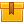# Codes, Encoders, Decoders, and Flip-Flops (A9402)

## Prerequisites

This lesson is designed for participants familiar with AC/DC theory, logic gates, and binary numbering systems. A knowledge of pin connection diagrams and wiring diagrams is also required.

## Description

This lesson discusses codes, encoders, and decoders and explains how to troubleshoot these circuits. In addition, the lesson addresses the operation and troubleshooting of flip-flops.

## Objectives

Convert between BCD and decimal numbers. Identify and describe the function of active low inputs and outputs. Explain the functions of pins on an encoder and the operation of a seven segment LED display. Determine whether encoder and decoder circuits are functioning properly. State the difference between combinational and sequential logic circuits. Describe the operation of R-S flip-flops, D flip-flops, and J-K flip- flops. Describe the operation of an IC latch. Interpret flip-flop truth tables. Determine whether flip-flop circuits are functioning properly.

## ContentThis lesson discusses codes, encoders, and decoders and explains how to
•Codes, Encoders, Decoders, and Flip-Flops